# Euler problem 62

The cube, 41063625 (3453), can be permuted to produce two other cubes: 56623104 (3843) and 66430125 (4053). In fact, 41063625 is the smallest cube which has exactly three permutations of its digits which are also cube.

Find the smallest cube for which exactly five permutations of its digits are cube.

Alright. I need to find five cubes, that are permutations of the same digits.

How to check if two numbers are permutations of each other?

We can generate the largest permutation of a given number. If the largest permutation of two numbers are identical, the two numbers are permutations of each other.

So I need a function, that returns the largest permutation of a number. It would be nice, if that function was vectorized.

``````max_perm <- function(t){
require(magrittr)
options(scipen=5)
t %>%
as.character() %>%
str_split("") %>%
lapply(sort, decreasing=TRUE) %>%
lapply(paste0, collapse="") %>%
unlist() %>%
as.double()
}
``````

Convert the input to character. Split at “”. That returns a list with vectors containing the individual digits of the input. lapply sorts the individual vectors in the list in decreasing order. Then lapply pastes the elements in each vector together with paste0 and “” as the separator. Then it is unlisted, and returned as numeric.

What is worth noting is a thing I was struggling with for far too long. R likes to write numbers in scientific notation. As in “1e+06”. I have not studied the phenomenon in detail. But options(scipen=5) solves the problem. It is the “penalty” used to decide when a number should be written in scientific notation. Unless I change that (trial and error, but it should be larger than whatever is default), as.character(1000000) will return “1e+06”. And the permutations of “1” “e” “+” “0” “6” are not terribly useful in this context.

I’m hazarding a guess that I don’t need to handle cubes of values of more than four digits.

Beginning with a vector of all numbers from 1 to 9999, I convert it to a dataframe. I transmute the first column to a column with the name x.
Then I mutate a second column, cube, into existence, and calculate it as the cube of the x-value. A third column, max_cube, is mutated with the result from my max_perm function above. And tha column is immediately used to group the data, so I get date grouped by identical maximum values of the permutations. I filter on the count of those groups, and only keep the groups that contain 5 elements. Then I ungroup it, and select just the cube column.

I now have a data frame with a single column containing 10 values. They are all cubes, five of them are permutations of each other. The other five are also permutaions of each other. And now I just have to take the smallest of them.

``````result <- 1:9999 %>%
as.double() %>%
as.data.frame() %>%
transmute(., x = .) %>%
mutate(cube = x**3) %>%
mutate(max_cube = max_perm(cube)) %>%
group_by(max_cube) %>%
filter(n()==5) %>%
ungroup() %>%
select(cube) %>%
min()
``````

Before I print the result, so I can enter it into Project Euler, I set options(digits=17).

Done! A good exercise. And a valuable lesson in the importance of the options in R.

# Waffle charts

A rather popular chart type. Not really my favorite, but I can see how it makes things easier to understand for people who are not used to read and understand charts. The reason for my less than favourable view on waffle charts are probably linked to its overuse in meaningless infographics.

A waffle chart is a grid with squares/cells/icons/whatever, where each cell represents a number of something.

Lets make an example:

``````library(ggplot2)
library(waffle)
``````
``````vec <- c(`Category 1 (10)`= 10 , `Category 2 (20)`= 20,
`Category 3 (25)`= 24, `Category 4 (16)` = 16)

waffle(vec/2, rows=3, size=0.1,
colors=c("#c7d4b6", "#a3aabd", "#a0d0de", "#97b5cf"),
title="Four different categories of something",
xlab="1 square = 2 somethings")
``````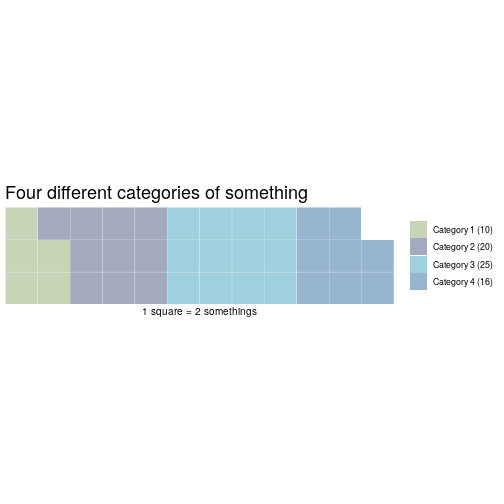One annoyance: waffle wants you to spell colours wrong.

waffle takes a named vector of values, rows sets the number of rows of blocks. Default is 10.

One standard way, is to show a 10×10 grid, where each cell represents 1% of the total:

``````waffle(vec/sum(vec)*100)
``````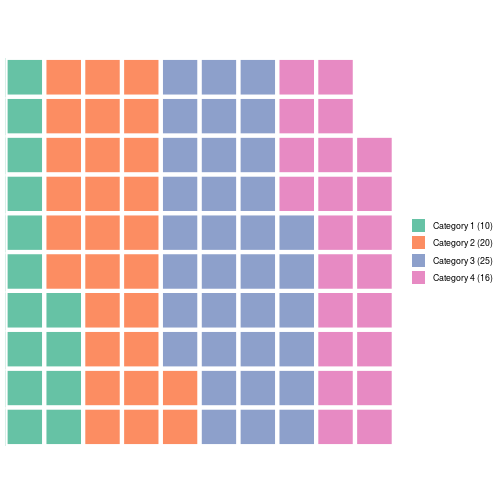Bloody annoying – waffle rounds the values of the vector, leading to only 98 squares. So you have to manipulate your vector to get to 100. Well, actually it is probably a minor annoyance.

What if you want something else than coloured squares?

The arguments “use_glyph” and “glyph_size” makes that possible.
First, we’ll need the library extrafont

``````library(extrafont)
``````

We’ll also need to have the “awesomefonts” installed. It can be downloaded from:

http://maxcdn.bootstrapcdn.com/font-awesome/4.3.0/fonts/fontawesome-webfont.ttf

This should be easier if you are on a desktop machine. As I’m running this through my own installation of RStudio on a remote server, it was a bit more difficult.

I needed to place the “fontawesome.ttf” file in the “/usr/share/fonts/truetype/fontawesome” directory.

Then, running R as superuser on the commandline, I imported the extrafont library, and then ran “font_import()”.

But then it worked!

There is now a long list of 593 different icons, that can be used. If you want a list, just run fa_list().

And now, we can make a waffle chart with the glyph of our choice.

``````waffle(vec/2, rows=4, use_glyph = "wifi")
``````
```## Warning: Removed 1 rows containing missing values (geom_text).
```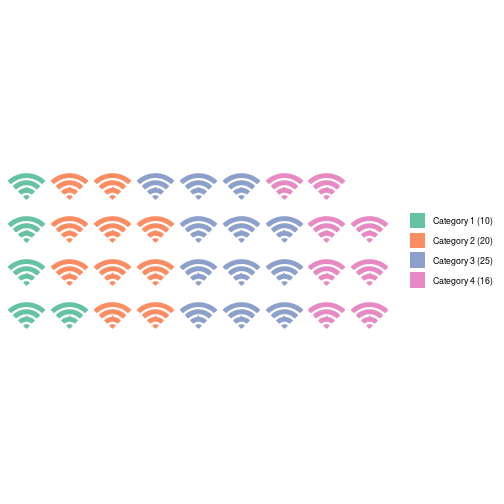We can change the colours:

``````library(RColorBrewer)
waffle(vec/2, rows=4, use_glyph = "wifi", colors=brewer.pal(4,"Set1"))
``````
```## Warning: Removed 1 rows containing missing values (geom_text).
```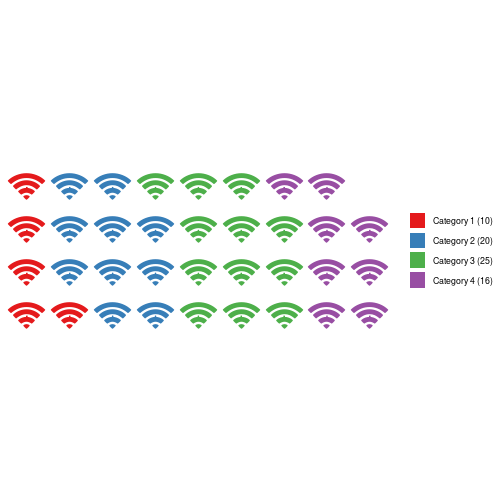``````waffle(vec/2, rows=4, use_glyph = "wifi", colors=brewer.pal(6,"Set1"), glyph_size=5)
``````
```## Warning: Removed 1 rows containing missing values (geom_text).
```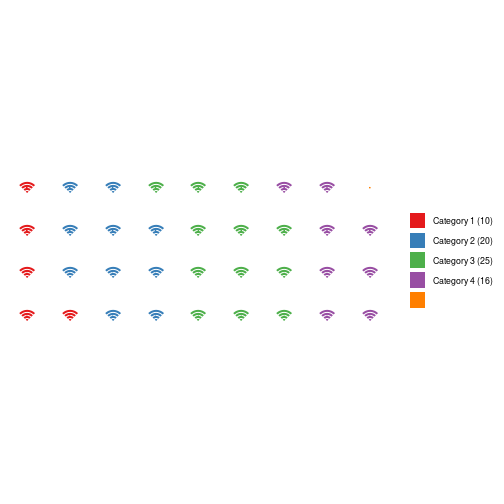But that does not look very good.

waffle is based on ggplot, so we have access to the full range of functionality. But not all of them are going to look good in this context:

``````waffle(vec/2, rows=4, use_glyph = "wifi", colors=brewer.pal(4,"Set1")) +
geom_label(label="42", size = 3)
``````
```## Warning: Removed 1 rows containing missing values (geom_text).
```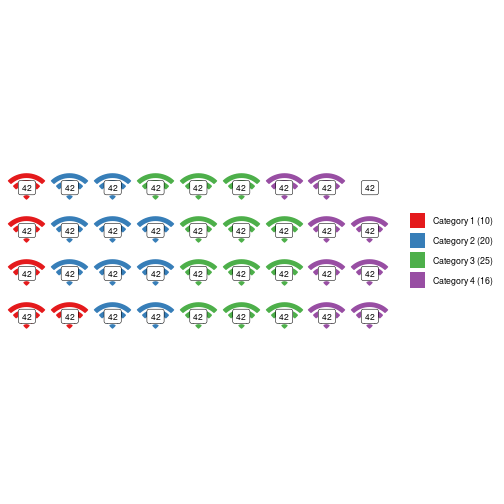If we install a font that supports it, we even get access to the large number of UTF-8 glyphs. Here is a favorite of mine:

``````waffle(vec/2, rows=4, colors=brewer.pal(4,"Set1")) +
geom_label(label=sprintf("\U1F427"), size = 8)
``````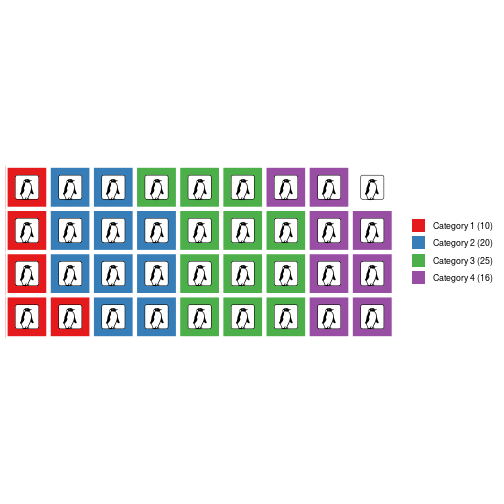Which of course requires you to have a font on your computer that supports penguins.

Here is one:
http://users.teilar.gr/~g1951d/Symbola.zip

Udgivet i R

# Get the font color of a cell in Excel

People do weird and wonderful things in Excel.

Other people then have to pull out the data from those spreadsheets.

“Other people”  tend to spend a lot of time crying into their coffee.

At the moment, I am trying to pull out data of a spreadsheet, where “something” can have a value of 1, 2 or 3. That is of course marked by an “x” in a cell. I need to convert that x to a number.

That is rather simple. What is not so simple, is that there can be two x’es. One, in black, to denote the current state of affairs. And a second x, in red, to denote what a future, state is wanted to be.

So – I need a way to get the color of an x. VBA can do that:

Function GetColour(ByVal Target As Range) As Single
Application.Volatile
GetColour = Target.Font.Color
End Function

And if I need a logical test:

Function IsBlack(ByVal Target As Range) As Boolean
Application.Volatile
If Target.Font.Color = 0 Then
IsBlack = True
Else
IsBlack = False
End If
End Function